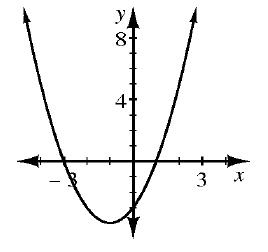### Home > CCAA8 > Chapter 9 Unit 10 > Lesson CCA: 9.1.3 > Problem9-29

9-29.

Find the equation that represents the information given below.

1.If $y=\left(x-5\right)\left(x+7\right)$, then the $x$-intercepts of the graph are $\left(5,0\right)$ and $\left(-7,0\right)$.
Use the $x$-intercepts of each representation to write your equations.

$y=\left(x+3\right)\left(x-1\right)$

1.  $x$ $y$ $-4$ $-3$ $-2$ $-1$ $0$ $1$ $2$ $3$ $4$ $12$ $5$ $0$ $-3$ $-4$ $-3$ $0$ $5$ $12$